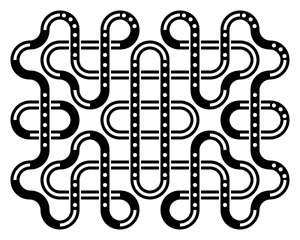# Is It Possible To Use Random Extension Using Percentage?

So I Was Planning on Making an Unnamed Game, And Figured Randomization…
The Problem i Found is Using Random Extension Didn’t Allow Percentage Like 5%…
I Was Hopefully Using it For A Simulation game… Any Work arounds?

1 Like

Can you use decimals like .05 for 5%? Maybe I’m misunderstanding.

The Way Random Extension Works is You Make a First Number and Lastnumber ex (1,5) then the extension will Choose either 1 - 5(any in between), i want to somehow… hopefully use percentage
since i want an uneven randomize, instead of all having same amount of percentage… and sure i know you could in theory use 1-3 and 4-5 so that would make it 70% but still… I am looking for ease use(hopefully) rather than having 2 more function

I think I understand. You want a little controlled randomness. A little bias so some numbers come up more often.

I was trying to recall where I used something like that. I made a Truchet Tile drawing app in Visual Studio bc I’m also into drawing. I wanted to add some bias to the tiles that it randomly picked. So, say a straight piece came up more frequently. I created a pool of the tiles I wanted to use and then repeated tiles that I wanted more frequently. So, I used an array with say “1,1,1,1,1,4,5,6” or “1,1,1,2,2,2,5,6,7,8” and then I picked a number the size of the array and then choose that element.

You can either “stack” the deck or do something if the number is in a range, say if it’s between 3 and 10 then do something.3 Likes

Sounds like what you’re after is random numbers with weightings. Here’s a post from a while back that mentions it, and a possible way to achieve it (pretty much what @Keith_1357 has described).

2 Likes

A percentage is commonly expressed in a scale of 0 to 100, so if what you want is as simple as a random percentage just use the random function with those parameters:

RandomInRange(0,100)

So, any value you get from it, that’s the random percentage.

BUT if you have predetermined values as the start and end points of, let’s say, a gauge and you want a random value inside that range, and at the same time you want to know the percentage it represents in the scale of your gauge… then you’re going to need to do a little bit more:

Let’s say the start value of your gauge is 1 and the end value is 6, so for getting a random value inside that scale you just need to do RandomInRange(1, 6). For this example, let’s say you got the random value 5,

… and now you want to know the percentage the random value 5 represents in the 1-6 scale, right? So, you’ve to multiply the random value (5) by 100 and then divide the result by the highest possible value in your scale (6), like this:

(5 × 100) ÷ 6 = 80

In the scale 1 to 6, the value 5 represents the 80% of it.

2 Likes

So like Basically(if i get this right) I will create an array with 1,5 then i could weight it down by let say (1, 1, 1, 2, 3, 4, 5) then i will randomized it from there? Im really sorry if i dont get it…

That’s the basic idea. That would give the #1 a 3 times odds advantage. Put numbers in array, choose a random element using random size of array.

Another way. Say you have 3 enemies. You want them mostly to be zombies.

Pick a random # from 1 to 10
If the number is <=7 then add zombie
If it’s 8 or 9 add vampire
If it’s 10 add a werewolf

That would basically be 70%, 20%, 10%

When I used the array idea, I had like 25 tiles to choose from. The array worked for me. It depends on your needed range.

1 Like

Alright thanks for the help, i really could’ve Fix this without you, Have a nice day

1 Like